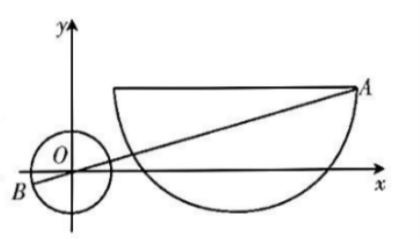(1)求曲线 $C$ 的普通方程和曲线 $M$ 的直角坐标方程;
(2) 若 $A, B$ 分别是曲线 $C$ 和曲线 $M$ 上的动点, 求 $|A B|$ 的最大值.
【答案】 解: (1) 因为 $\sin ^2 \theta+\cos ^2 \theta=1$, 所以 $(x-4)^2+(y-2)^2=9$,

(2) 由 (1) 知, 曲线 $C$ 为圆 $(x-4)^2+(y-2)^2=9$ 的下半部分,

$|A B|$ 可取得最大值, 且最大值为 $\sqrt{7^2+2^2}+1=\sqrt{53}+1$.